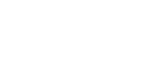ISSN 2542–0380 Труды Института механики им. Р.Р. Мавлютова Электронный научный журнал | Electronic Scientific Journal Proceedings of the Mavlyutov Institute of Mechanicsим. Р.Р. Мавлютова
УФИЦ РАНYulmukhametova Yu.V. Invariant submodels of a rank 2 with rotation for the equations of gas dynamics Proceedings of the Mavlyutov Institute of Mechanics. 10 (2014). 114–115.
2014. Vol. 10. Issue 1, Pp. 114–115
URL: http://proc.uimech.org/uim2014.1.022,en
DOI: 10.21662/uim2014.1.022
Invariant submodels of a rank 2 with rotation for the equations of gas dynamics
Yulmukhametova Yu.V.
Mavlyutov Institute of Mechanics, Ufa

### Abstract

The various invariant submodels of a rank 2 allowing the operator of rotation for the equations of gas dynamics with the arbitrariest equation of state are considered. It is shown that such submodels come down to an ordinary differential equation of the second order.